|

# 梁志天新品“宁静的奢华”入驻C&U香奈宜居国际家居

6月15日，香奈宜居国际家居品牌所代理的梁志天「宁静的奢华」系列新品于2018北京国际家居展暨中国生活节在北京·中国国际展览中心(新馆)W1馆C07展厅隆重发布。现场梁志天带来了「宁静的奢华」系列新品，凭借Theodore Alexander (TA) 悠久的品牌历史和精湛的工艺，「宁静的奢华」展现出恰到好处、历久不衰的优雅。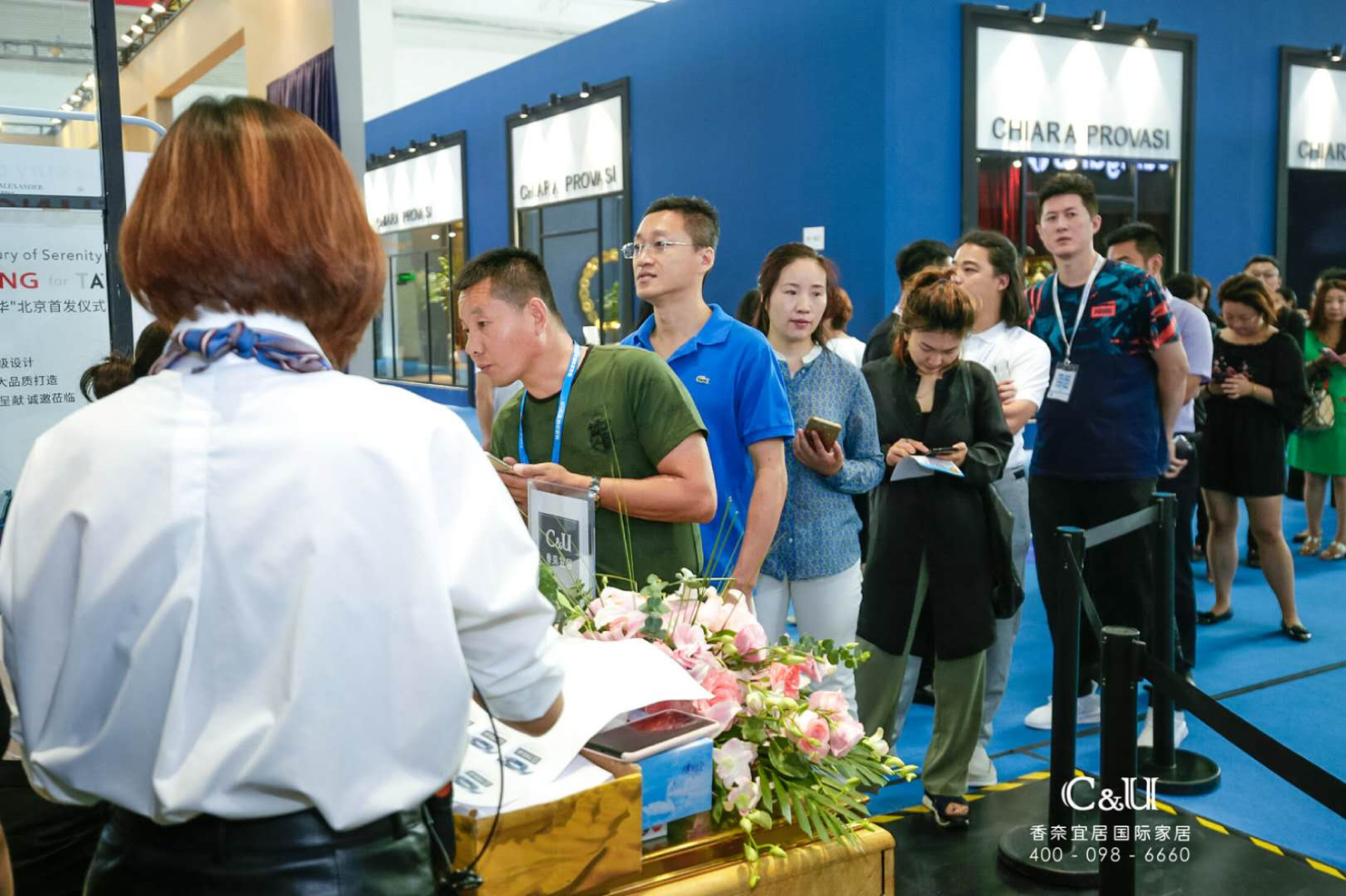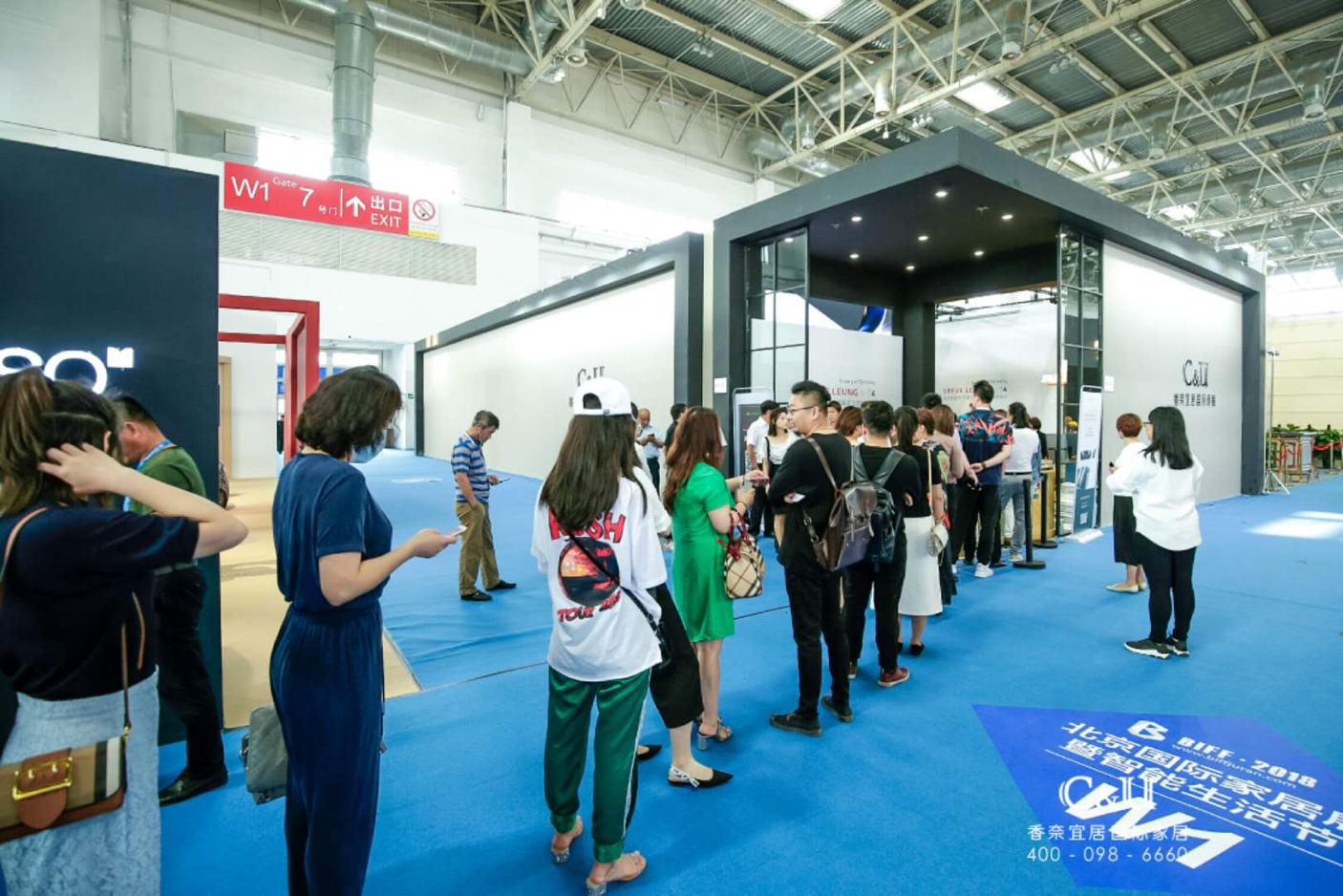国际室内建筑师/设计师团体联盟（IFI）2017-2019年度主席，SLD梁志天设计师有限公司创始人梁志天先生、香奈宜居国际家居董事长万飞燕女士、居然之家常务副总裁任成、中创意家居股份有限公司CEO、国际著名家具品牌---西奥多·亚历山大中国区总裁黄志新先生，共合设联合创始人吴巍、共合设联合创始人金永生、孟也空间创意设计事务所设计总监、渡道国际空间(北京)设计创始人--孟也，以及来自全国的各家新闻媒体出席本次新品发布会，共同见证了香奈宜居国际家居品牌合作梁志天系列新品北京首发的具有历史意义的时刻。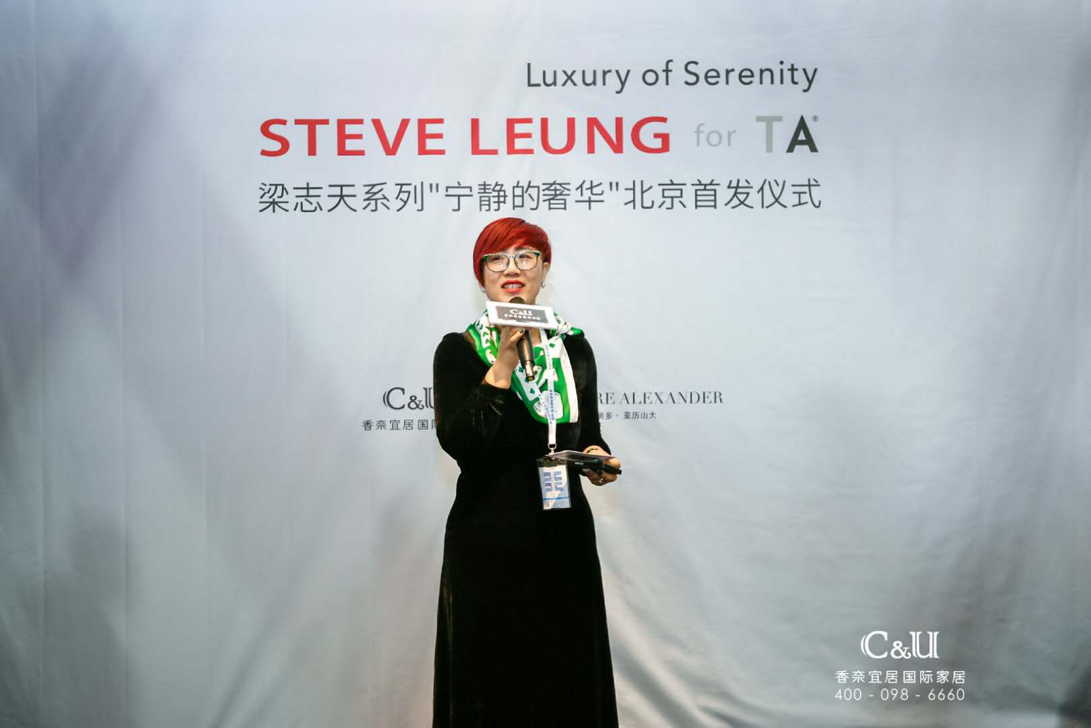赵娜女士致辞介绍，香奈宜居国际家居的品牌主张是缔造美好生活，作为高品质家具的引领者，整合众多高端商品与优秀设计师，为客户提供包括-家具、窗帘、地毯、灯饰、油画、饰品、床品、卫浴等高端软装，将各种高品质风格的生活情境做实体展演，做整体结合，以情境式展示呈现各式生活概念与生活型态，给客户提供全套完整的高端软装执行方案。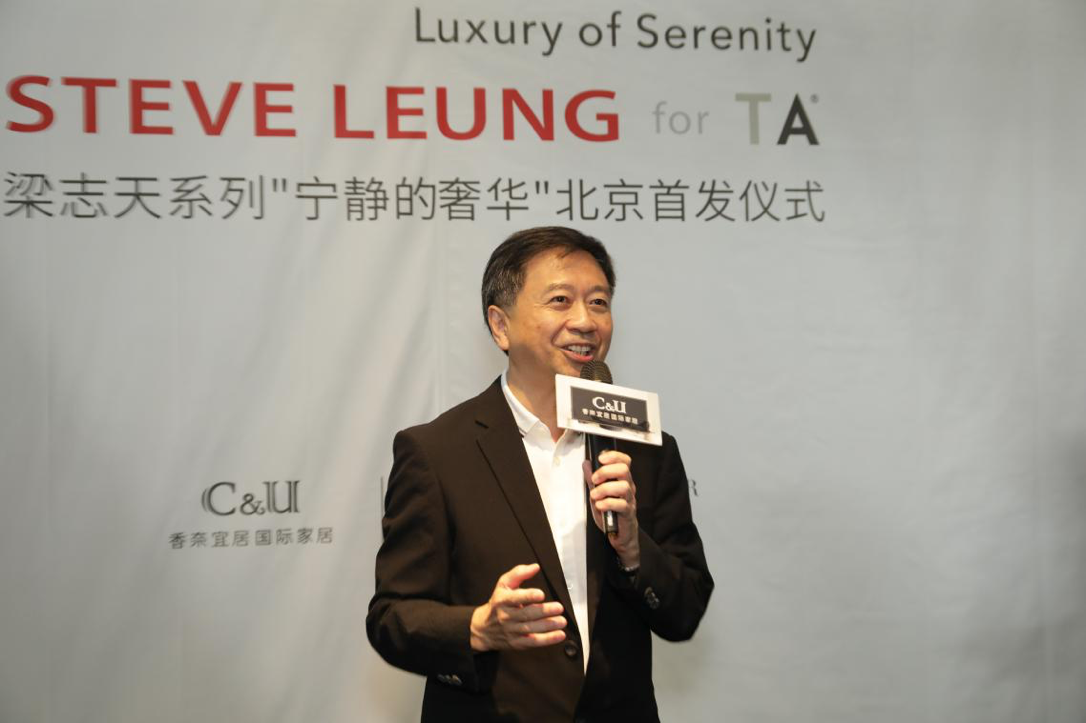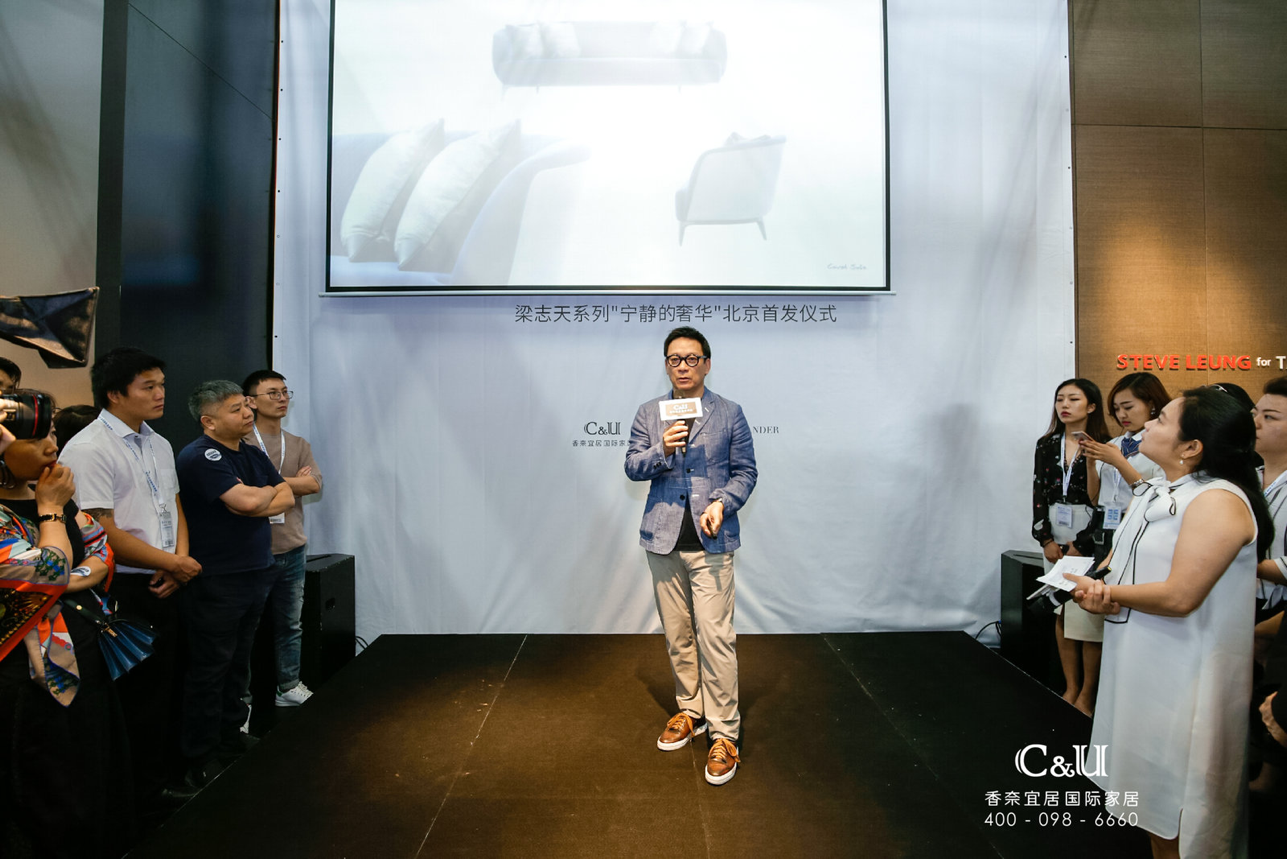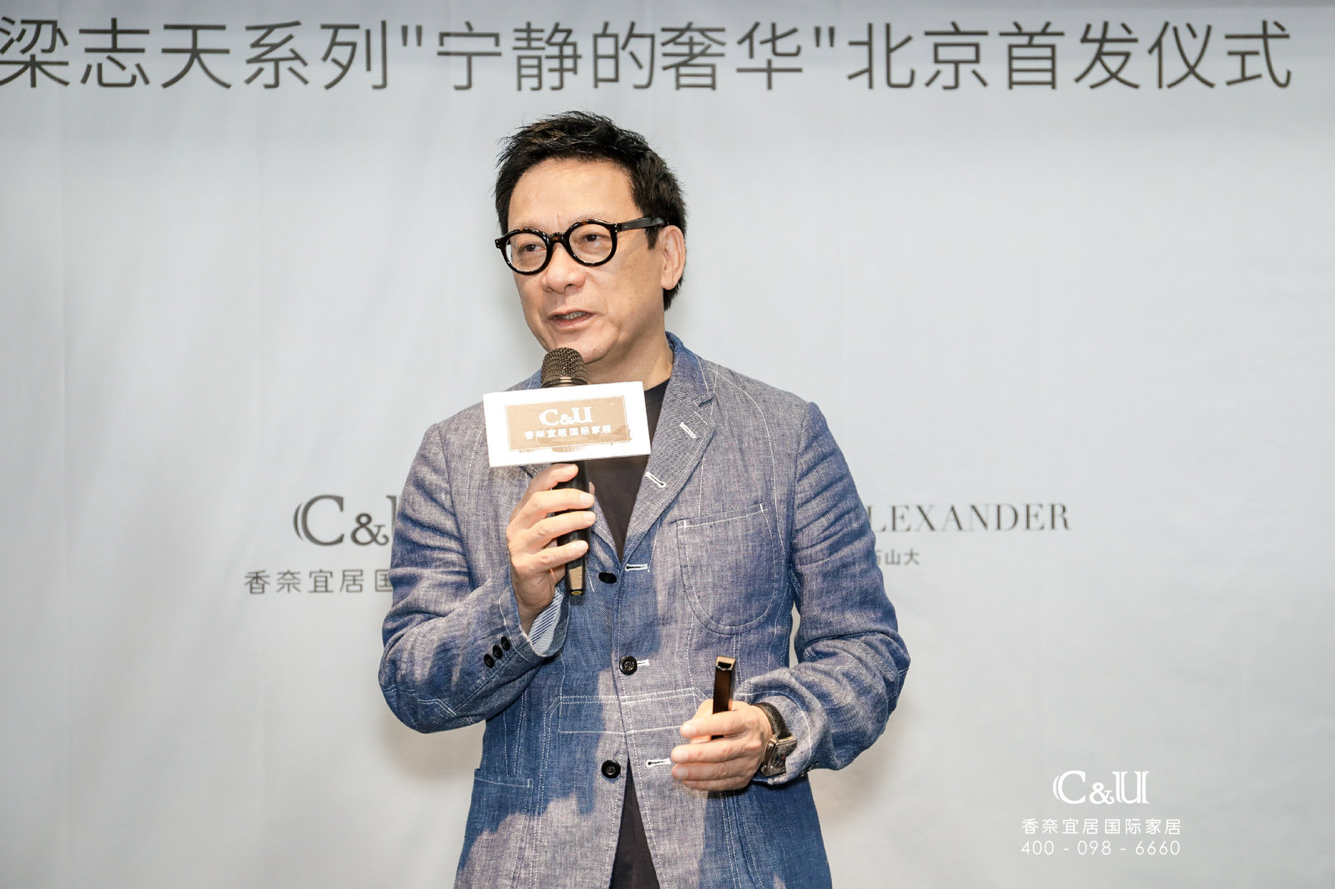国际室内建筑师/设计师团体联盟（IFI）2017-2019年度主席，SLD梁志天设计师有限公司创始人梁志天先生

梁志天“宁静的奢华”系列新品家具亮相新品发布会

新品发布会上，万飞燕女士，居然之家常务副总裁任成，创意家居CEO/西奥多·亚历山大中国区总裁黄志新先生，梁志天先生，共合设联合创始人吴巍，共合设联合创始人金永生，孟也空间创意设计事务所设计总监、渡道国际空间(北京)设计创始人--孟也一起共同为新品剪彩。本次发布会上，香奈宜居国际家居带来了梁志天系列「宁静的奢华」的新品。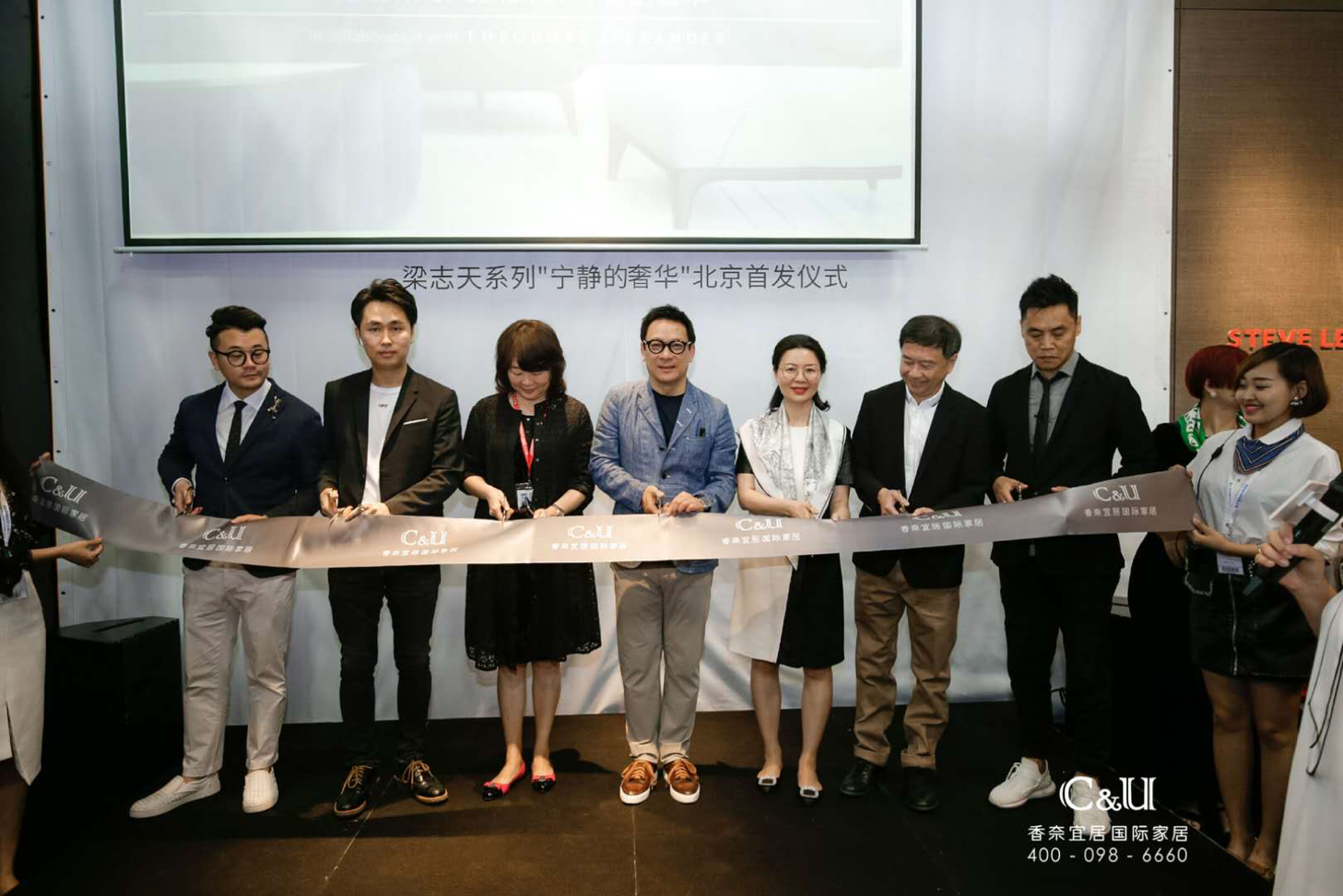「宁静的奢华」旨在探究个人极致的生活享受。整个系列以细致的线条贯穿，配以精湛的工艺和家具独特的流线形态，椅背的设计更让人感到放松安心，系列从细节中展现高贵的气息，为现代繁忙的生活，缔造具质感、温暖、宁静且高雅的空间。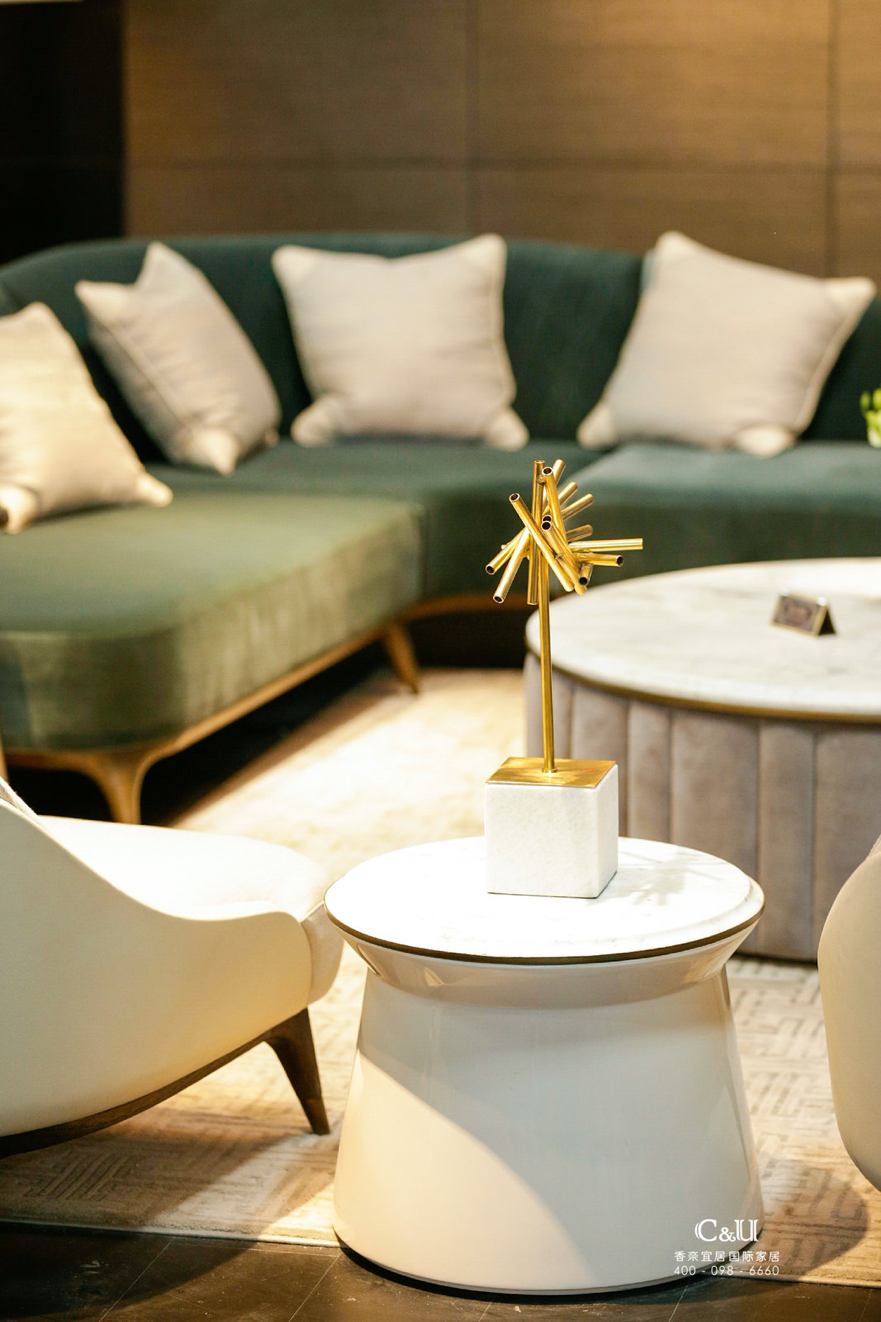Covet –Sectional Sofa美丽的流线型设计配以大小适中的靠背和组合式茶几，营造出一个轻松的氛围。以两色调作设计，配以菱形天鹅绒、皮革坐椅、木质底和铜脚。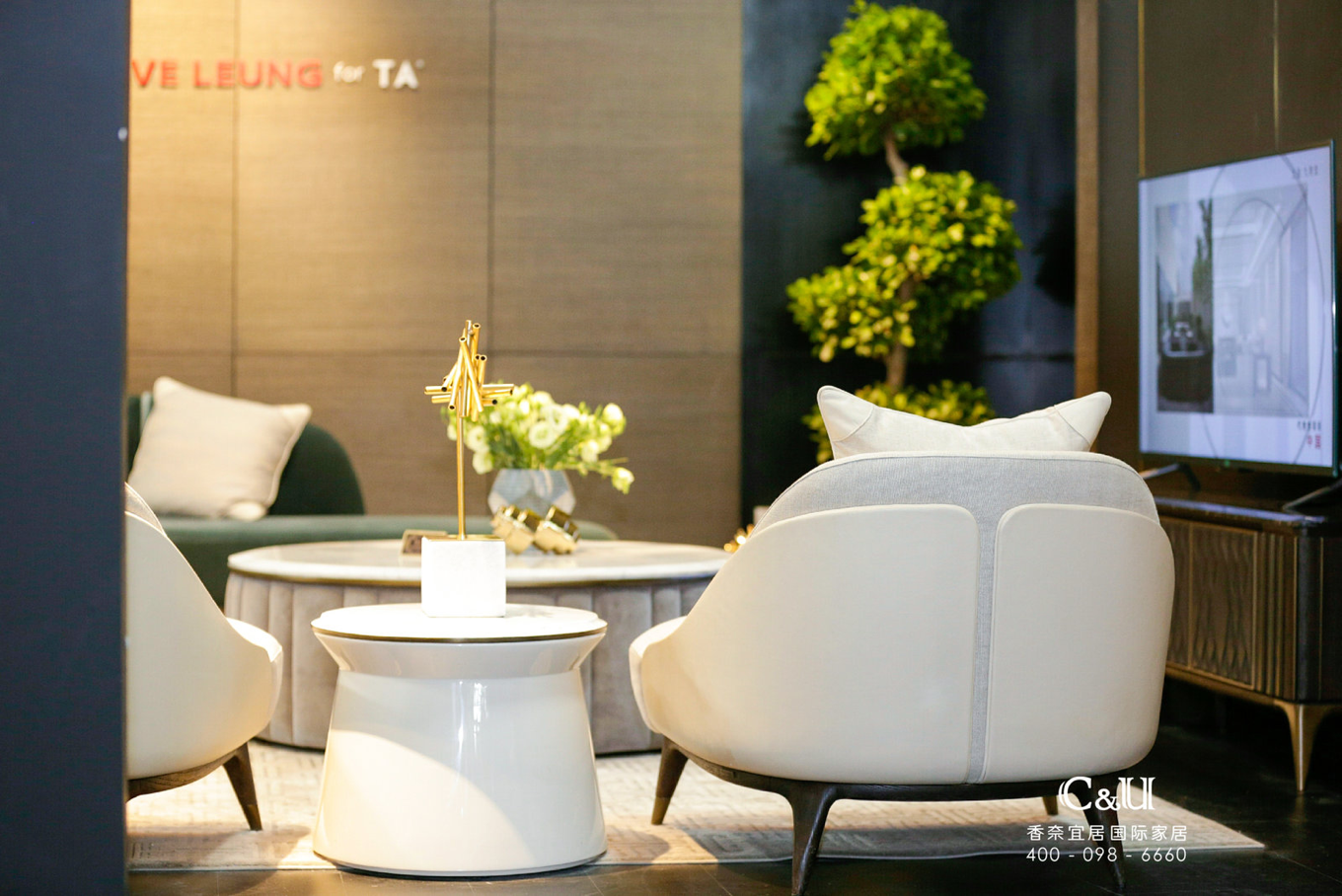Covet Deep Desire - Armchair以皮革设计，使用家拥入柔软的的天鹅绒里，缔造一个温暖、舒适的享受。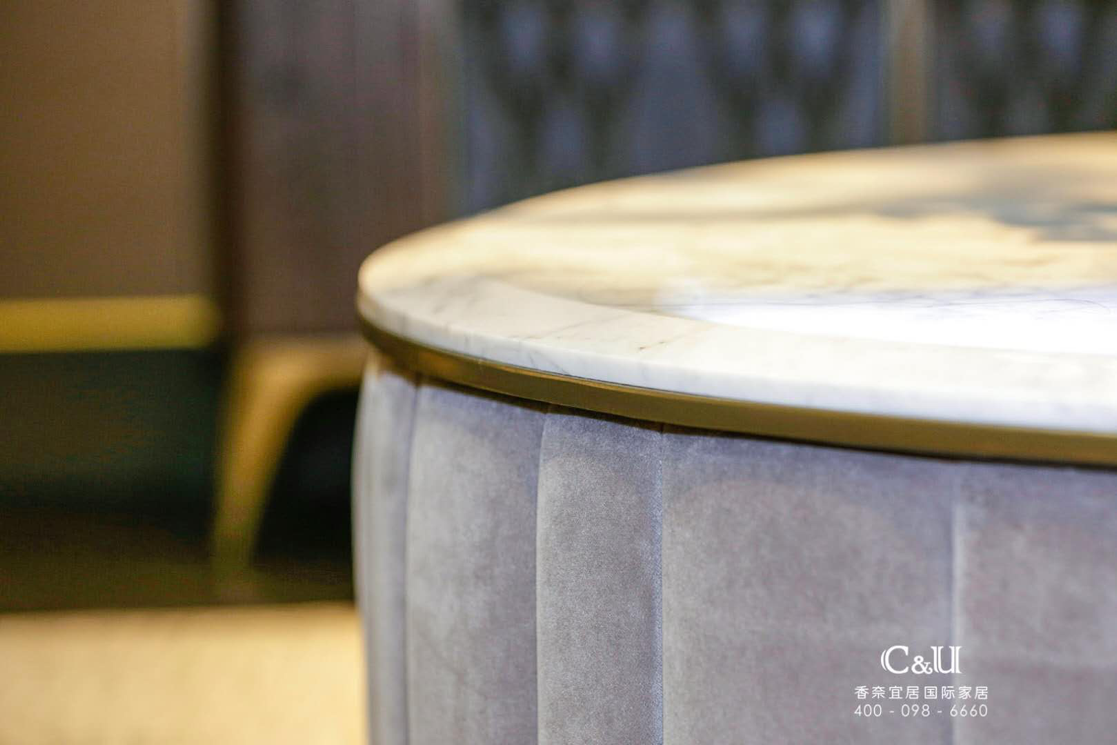Allure Attraction - Coffee table优美、柔软的罗纹设计吸引用家亲手感受大理石面，欣赏其恰到好处的打磨技术。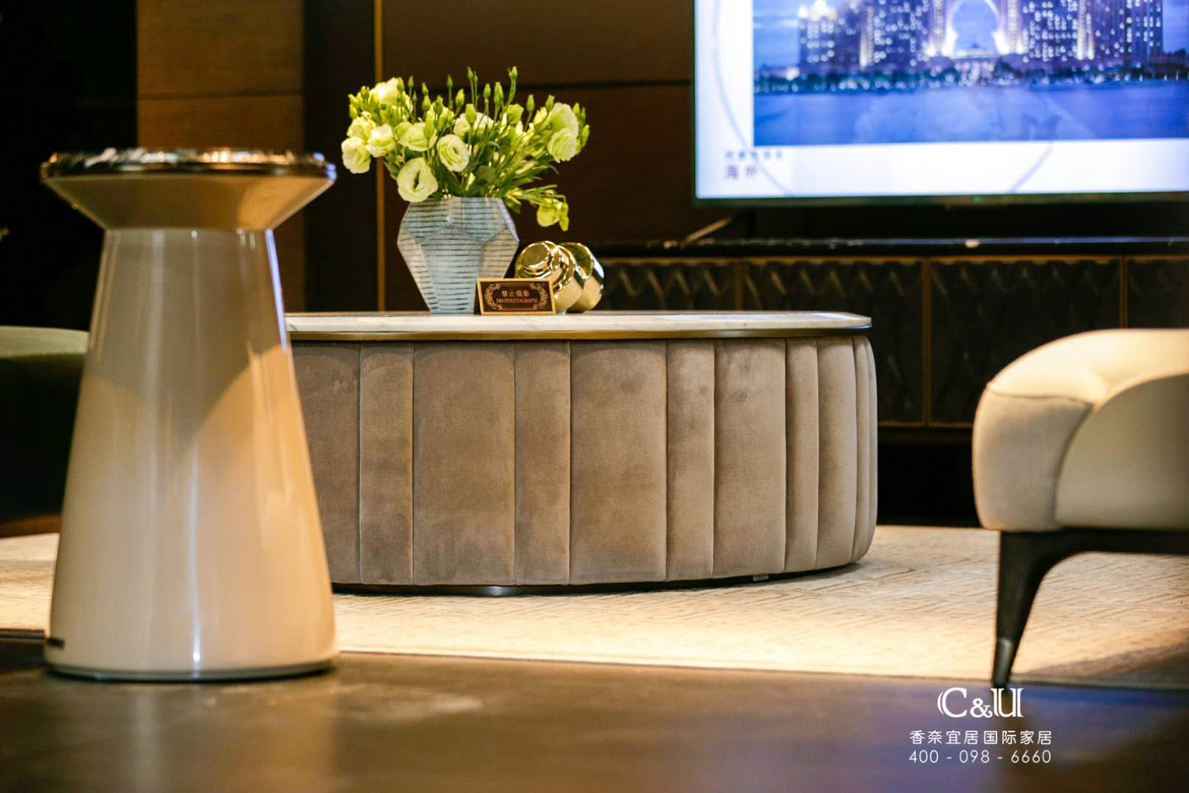Contour - Side table small轻圆的线条打造出一个与别不同、沉隐內斂的中式色彩茶几。油漆饰面配以镶著铜框的大理石面。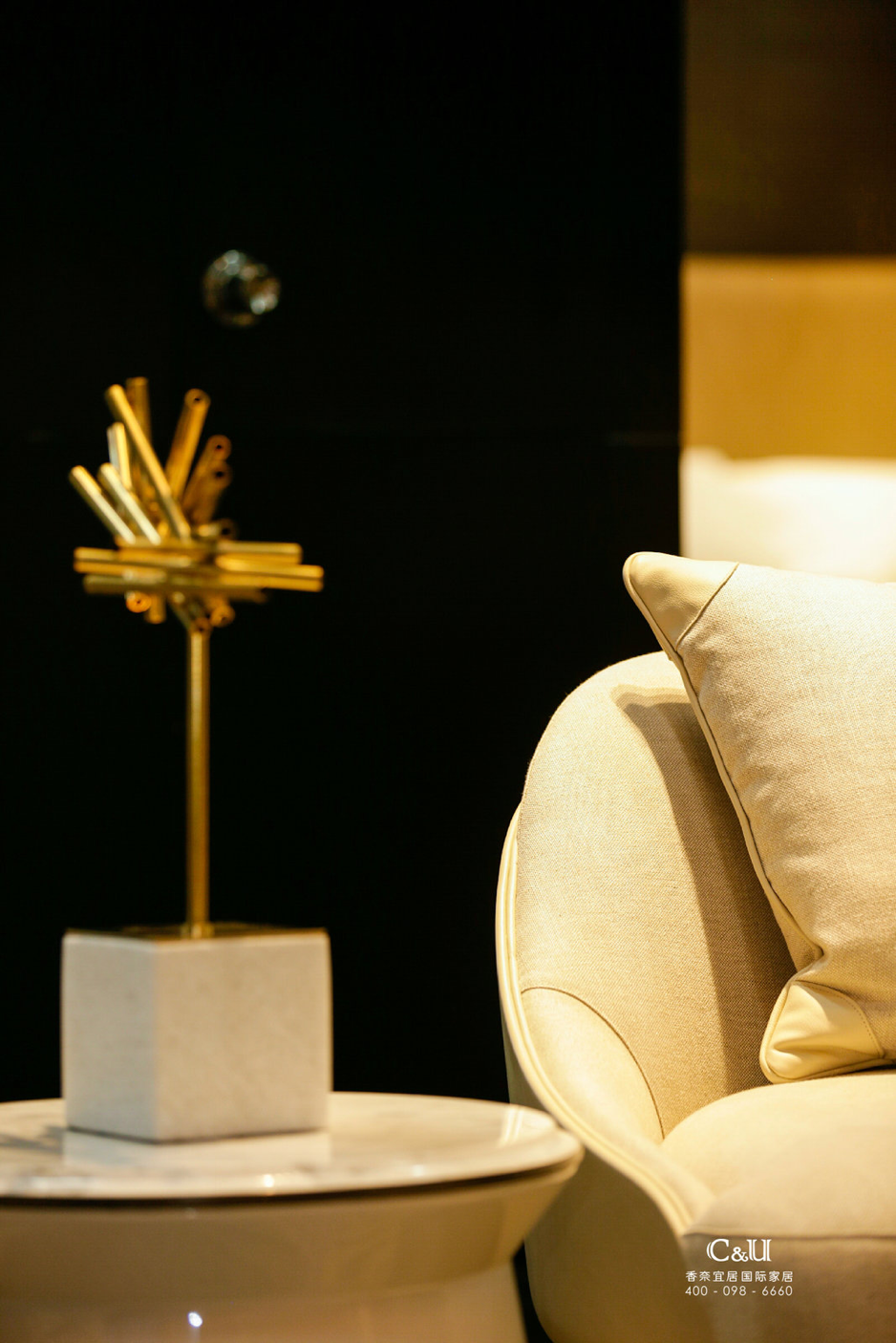Contour - Side table large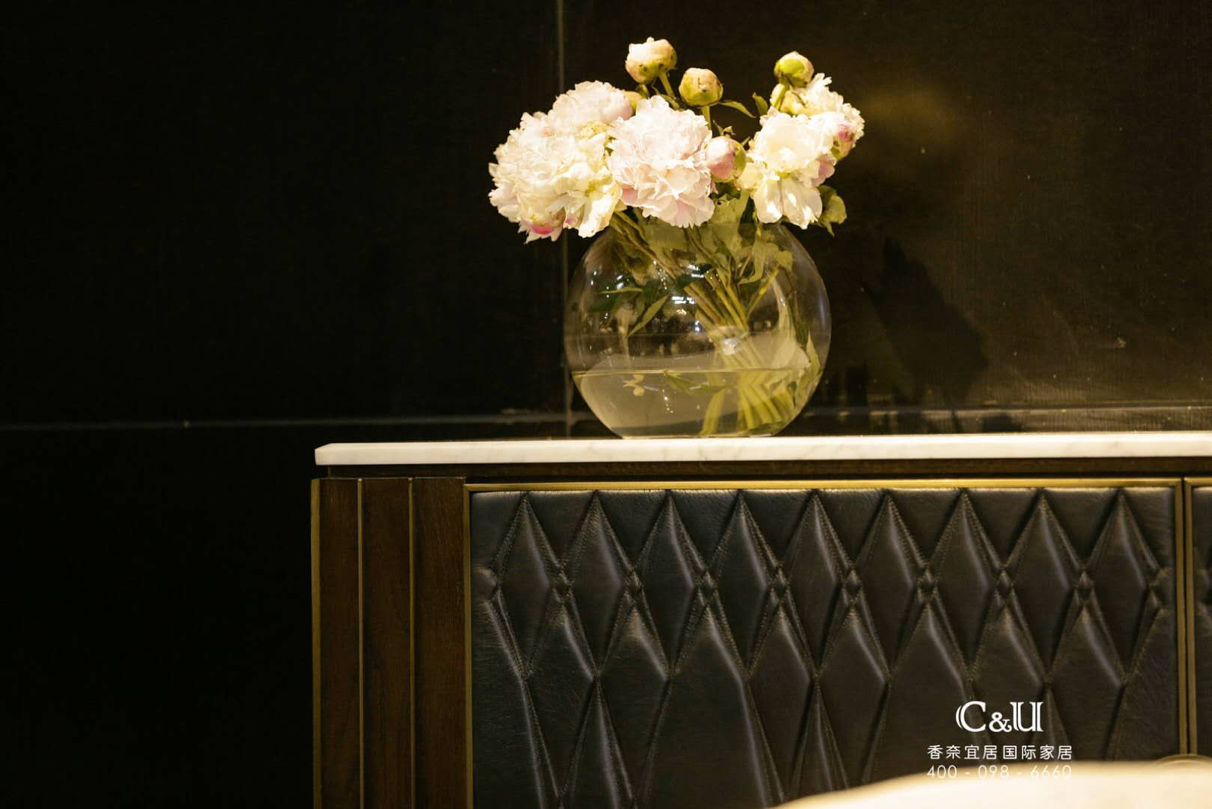Connoisseur –TV Cabinet优雅得体的大理石柜深得电视及配件喜好家欢心，又能用其展示独特的收藏品。菱形皮革门配以金属特色配件。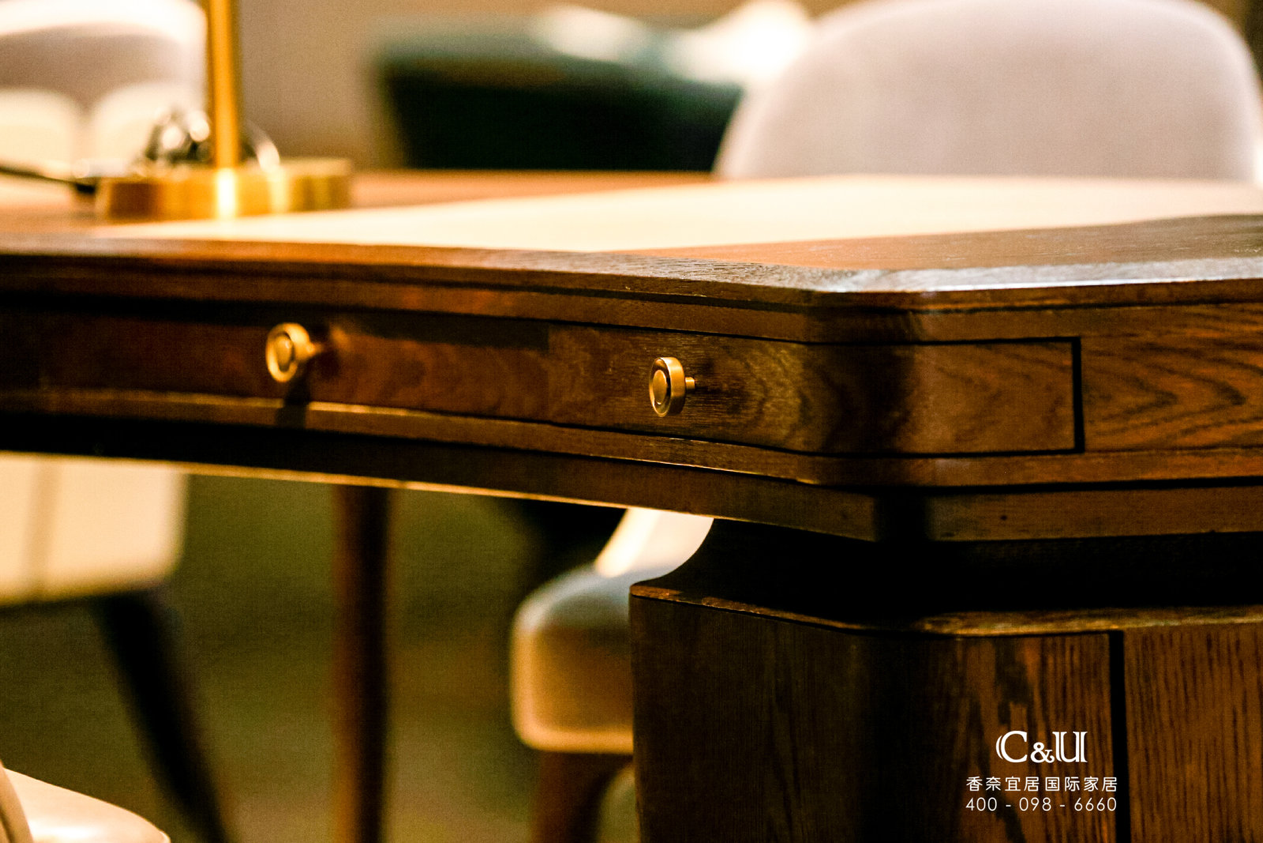Legacy –Pedestal Desk弯曲形设计的写字台为写作和记录日常生活提供一个最佳的空间。Legacy有着标志性的台脚、三个细长的抽屉以及皮革饰面的书写位置。Presence –Executive chair充滿威嚴的皮革高椅，讓用家得以沉思。以皮革设计配以高靠背，Presence 行政座椅带来一个奢华、舒适的抚慰。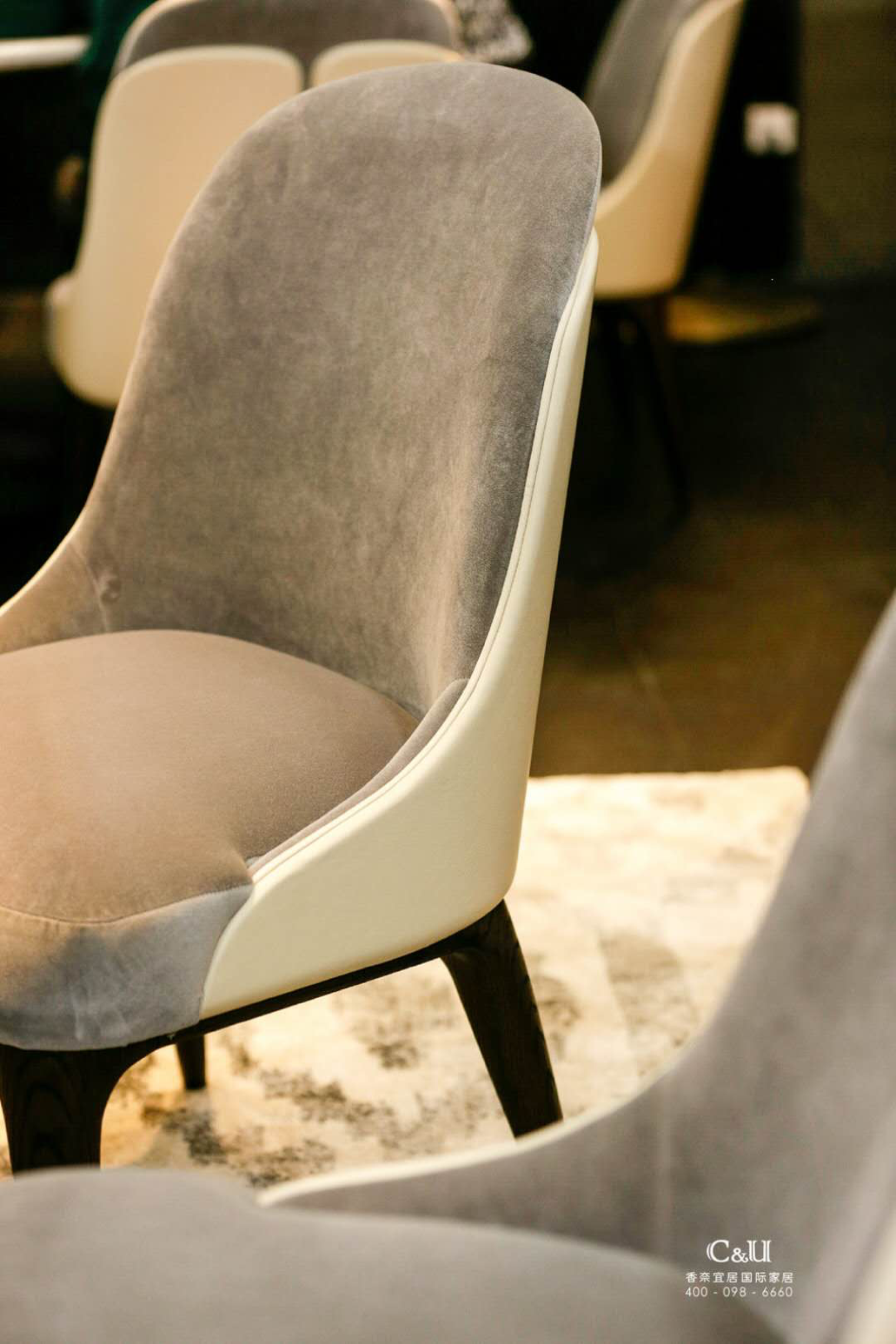Acquaint– visitor chair为匆匆来匆匆去的访客带来一个尊贵的地位和舒适的享受。秉承这系列，Acquaint以相互配合的两色为设计，配以皮革和布料坐椅。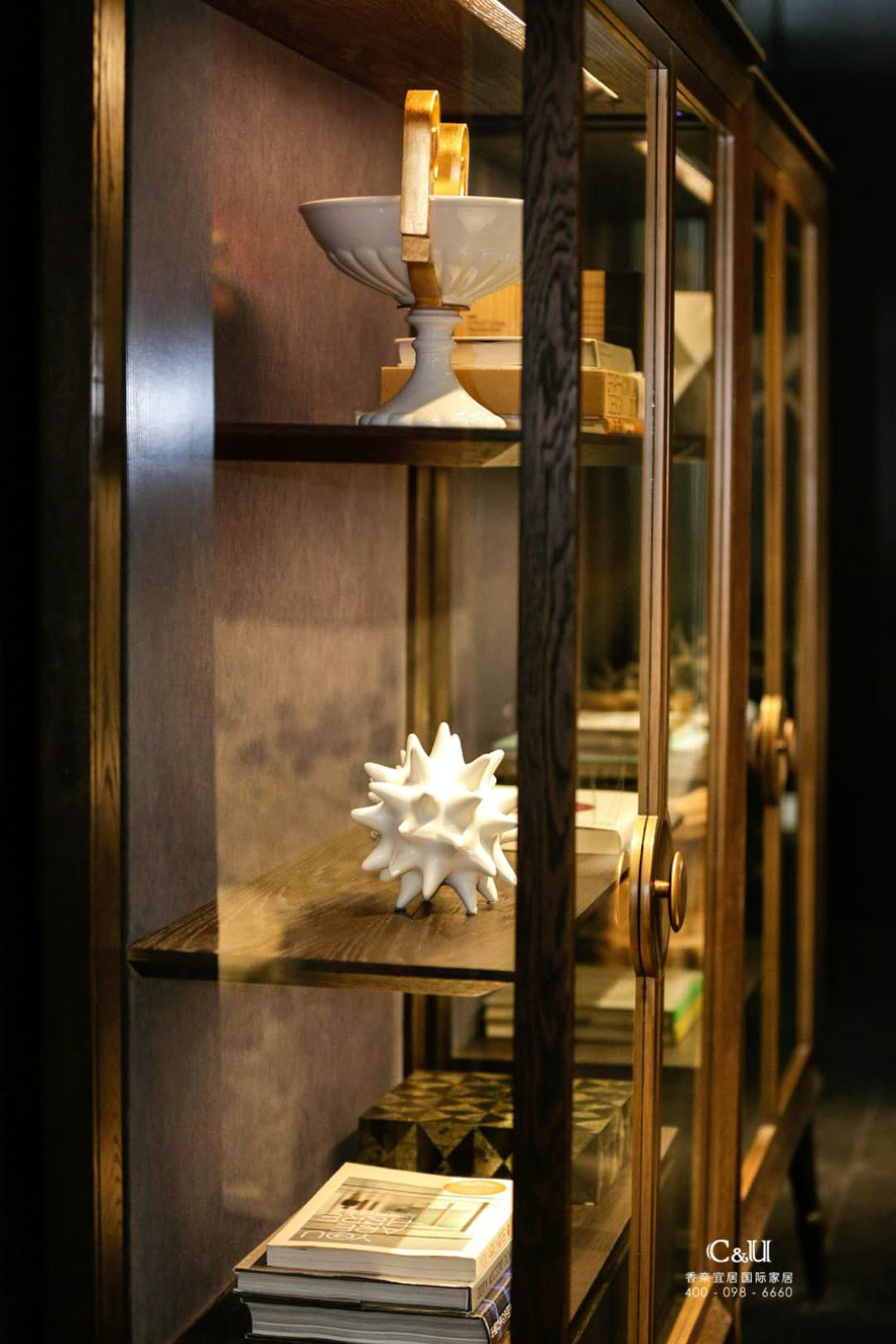Athenaeum –book case让用家在轻松的环境下，一边享用美酒，一边于火炉旁阅读。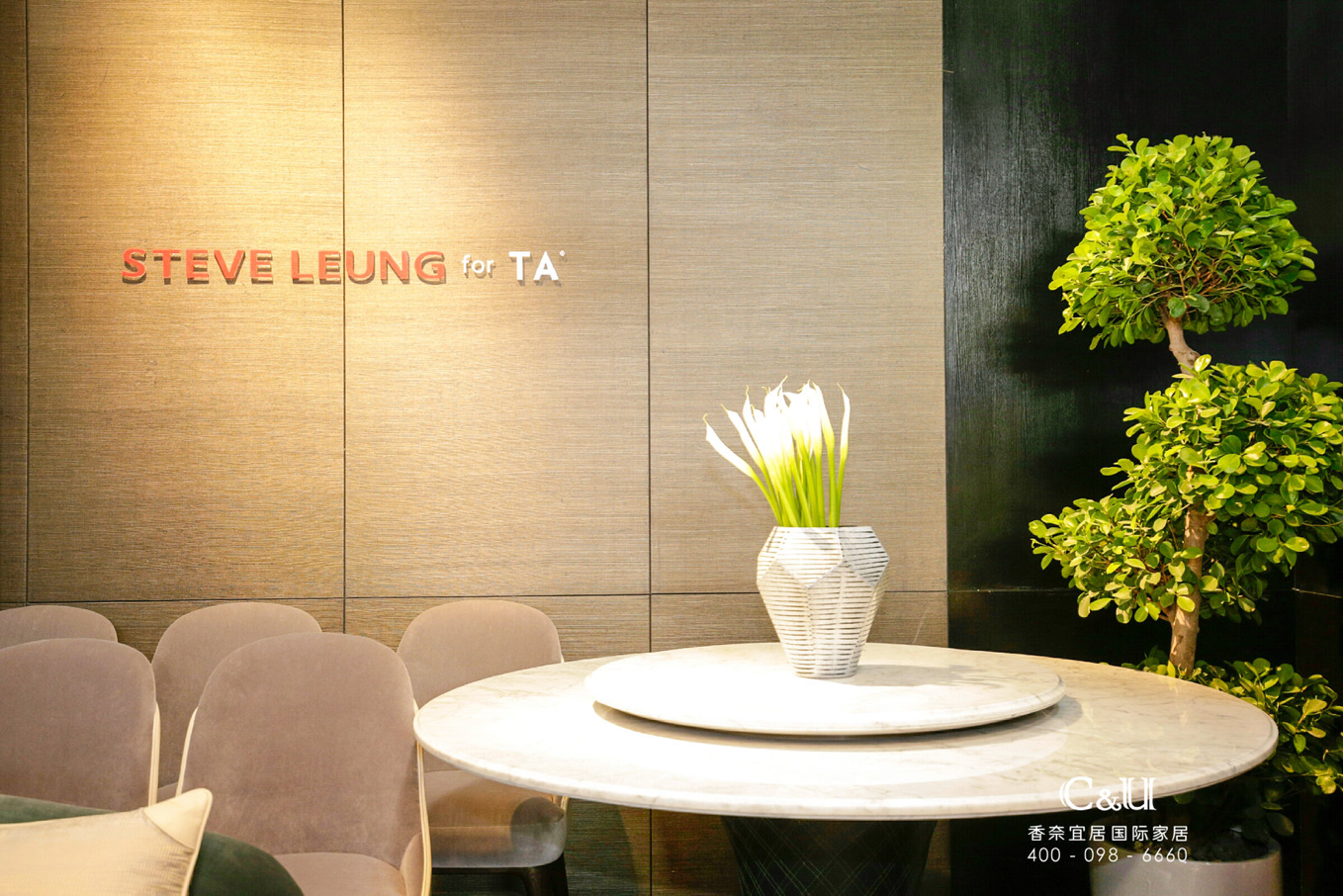Pirouette –Round Dining table大理石台面配以皮革设计的台身，其旋菱形设计是参照芭蕾舞蹈员优雅的旋转动作。

现场，梁志天还与多名知名设计师探讨家装设计对话。“嘈杂和烦躁的奢华，社会成长和发展，都驱使人们重新回归宁静生活！真正的奢华是适度、节制，与家人共享生活。消费者的认同不仅仅是对事物本身，而是背后体现的价值感。”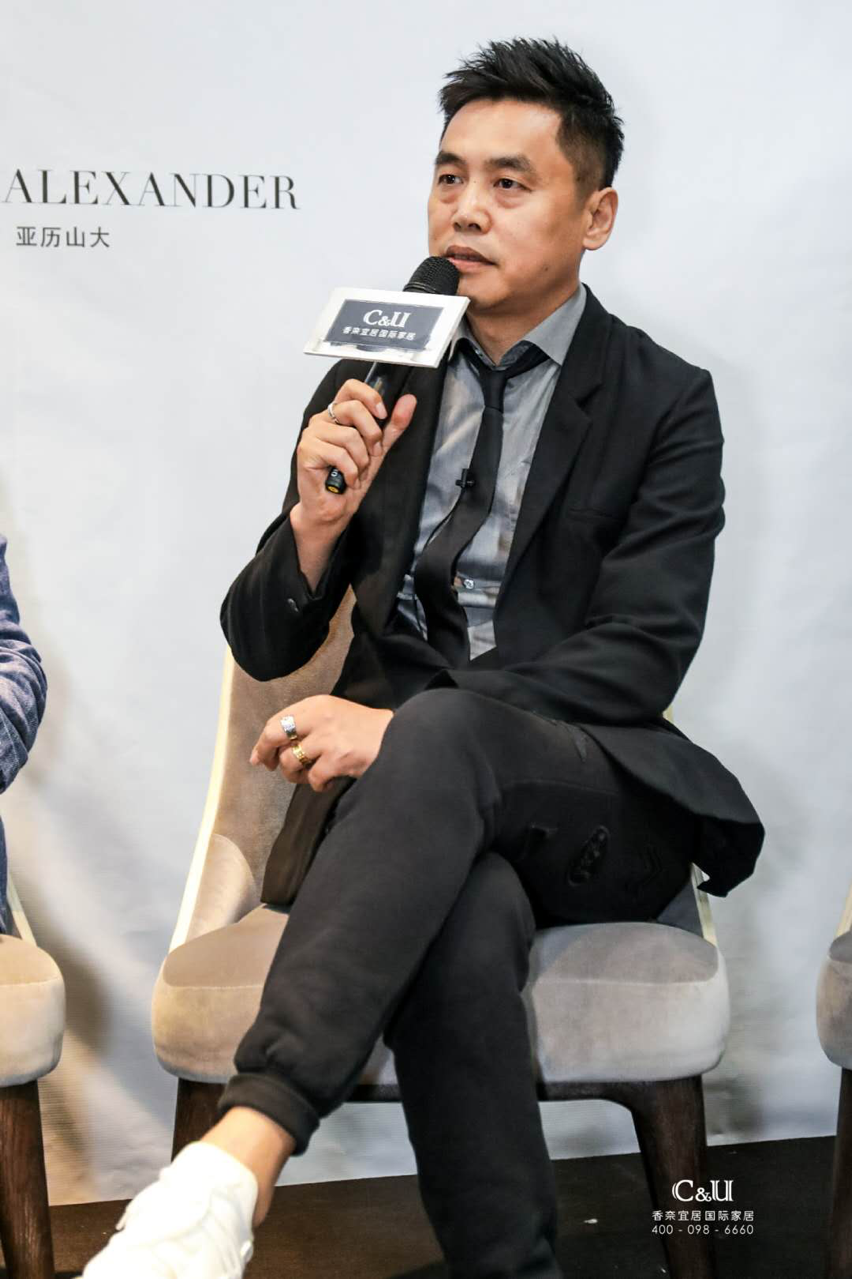“生活时代的发展和心境，都决定设计理念和状态。审美多样化，人生的不同阶段都有不同的选择。但梁老师的产品是国人追求的踏实，宁静。”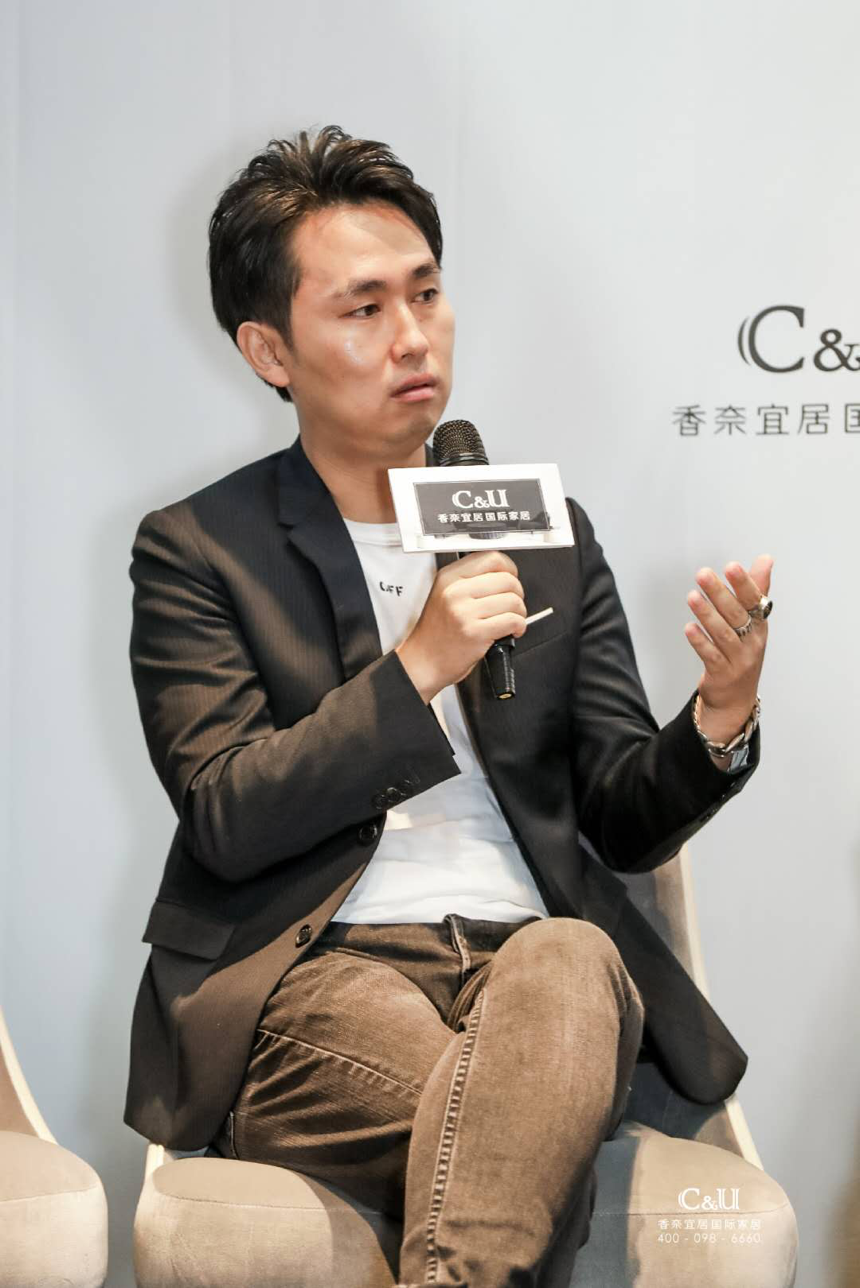“时间的脚印是会沉淀在设计上，梁老师的精益设计加上TA精湛工艺，打造强强联合的新品”。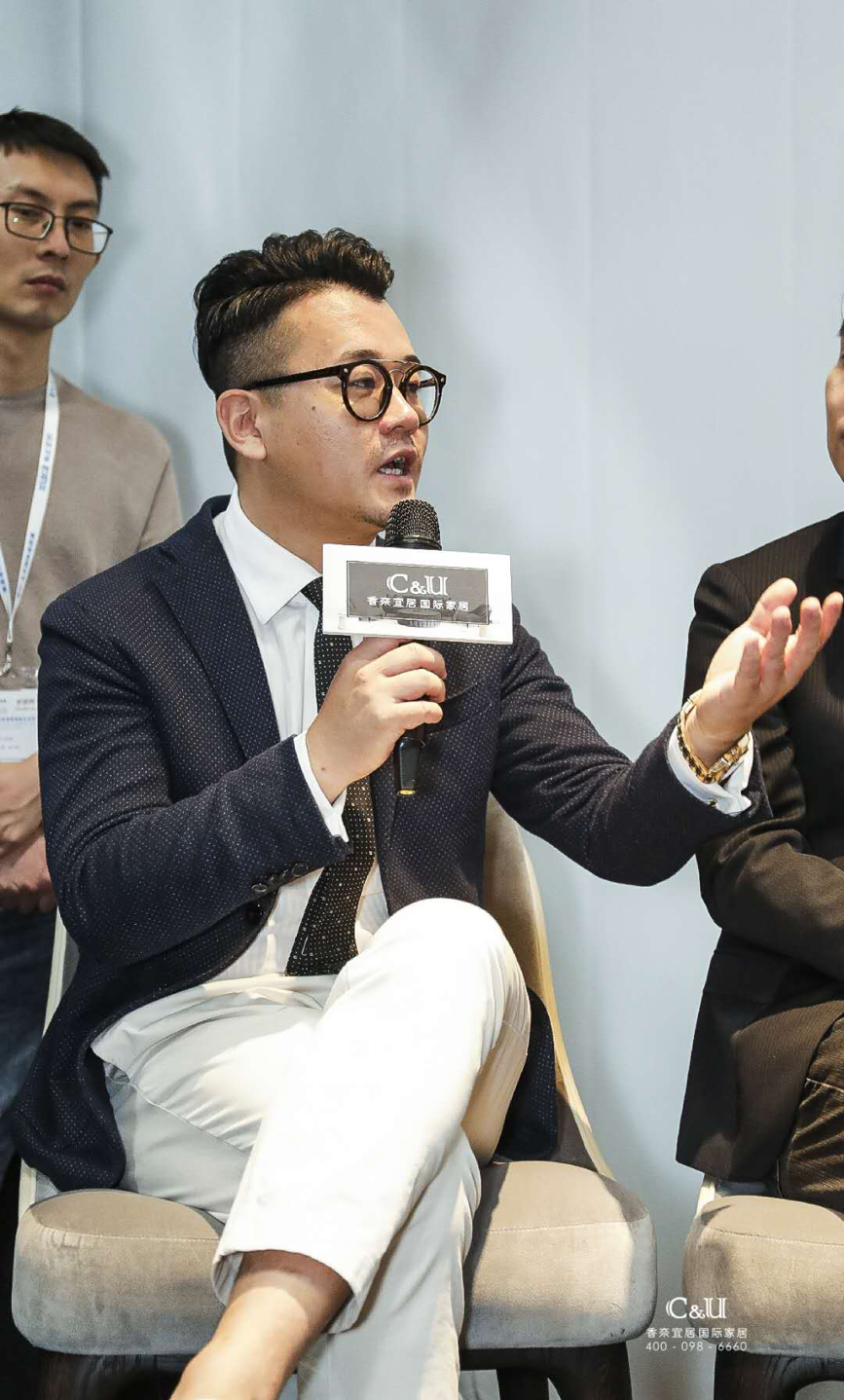香奈宜居国际家居作为中国家具软装全案设计代表的国际家居品牌，一直“视设计为企业灵魂”，坚持一贯秉承美好生活缔造者企业定位，正是这种多年的坚持，赢得了行业内外对香奈宜居的认可与肯定，参展作品一经亮相便获得广泛好评。

`声明：本文由入驻焦点开放平台的作者撰写，除焦点官方账号外，观点仅代表作者本人，不代表焦点立场错误信息举报电话： 400-099-0099，邮箱：jubao@vip.sohu.com，或点此进行意见反馈，或点此进行举报投诉。`A B C D E F G H J K L M N P Q R S T W X Y Z
A - B - C - D - E
• A
• 鞍山
• 安庆
• 安阳
• 安顺
• 安康
• 澳门
• B
• 北京
• 保定
• 包头
• 巴彦淖尔
• 本溪
• 蚌埠
• 亳州
• 滨州
• 北海
• 百色
• 巴中
• 毕节
• 保山
• 宝鸡
• 白银
• 巴州
• C
• 承德
• 沧州
• 长治
• 赤峰
• 朝阳
• 长春
• 常州
• 滁州
• 池州
• 长沙
• 常德
• 郴州
• 潮州
• 崇左
• 重庆
• 成都
• 楚雄
• 昌都
• 慈溪
• 常熟
• D
• 大同
• 大连
• 丹东
• 大庆
• 东营
• 德州
• 东莞
• 德阳
• 达州
• 大理
• 德宏
• 定西
• 儋州
• 东平
• E
• 鄂尔多斯
• 鄂州
• 恩施
F - G - H - I - J
• F
• 抚顺
• 阜新
• 阜阳
• 福州
• 抚州
• 佛山
• 防城港
• G
• 赣州
• 广州
• 桂林
• 贵港
• 广元
• 广安
• 贵阳
• 固原
• H
• 邯郸
• 衡水
• 呼和浩特
• 呼伦贝尔
• 葫芦岛
• 哈尔滨
• 黑河
• 淮安
• 杭州
• 湖州
• 合肥
• 淮南
• 淮北
• 黄山
• 菏泽
• 鹤壁
• 黄石
• 黄冈
• 衡阳
• 怀化
• 惠州
• 河源
• 贺州
• 河池
• 海口
• 红河
• 汉中
• 海东
• 怀来
• I
• J
• 晋中
• 锦州
• 吉林
• 鸡西
• 佳木斯
• 嘉兴
• 金华
• 景德镇
• 九江
• 吉安
• 济南
• 济宁
• 焦作
• 荆门
• 荆州
• 江门
• 揭阳
• 金昌
• 酒泉
• 嘉峪关
K - L - M - N - P
• K
• 开封
• 昆明
• 昆山
• L
• 廊坊
• 临汾
• 辽阳
• 连云港
• 丽水
• 六安
• 龙岩
• 莱芜
• 临沂
• 聊城
• 洛阳
• 漯河
• 娄底
• 柳州
• 来宾
• 泸州
• 乐山
• 六盘水
• 丽江
• 临沧
• 拉萨
• 林芝
• 兰州
• 陇南
• M
• 牡丹江
• 马鞍山
• 茂名
• 梅州
• 绵阳
• 眉山
• N
• 南京
• 南通
• 宁波
• 南平
• 宁德
• 南昌
• 南阳
• 南宁
• 内江
• 南充
• P
• 盘锦
• 莆田
• 平顶山
• 濮阳
• 攀枝花
• 普洱
• 平凉
Q - R - S - T - W
• Q
• 秦皇岛
• 齐齐哈尔
• 衢州
• 泉州
• 青岛
• 清远
• 钦州
• 黔南
• 曲靖
• 庆阳
• R
• 日照
• 日喀则
• S
• 石家庄
• 沈阳
• 双鸭山
• 绥化
• 上海
• 苏州
• 宿迁
• 绍兴
• 宿州
• 三明
• 上饶
• 三门峡
• 商丘
• 十堰
• 随州
• 邵阳
• 韶关
• 深圳
• 汕头
• 汕尾
• 三亚
• 三沙
• 遂宁
• 山南
• 商洛
• 石嘴山
• T
• 天津
• 唐山
• 太原
• 通辽
• 铁岭
• 泰州
• 台州
• 铜陵
• 泰安
• 铜仁
• 铜川
• 天水
• 天门
• W
• 乌海
• 乌兰察布
• 无锡
• 温州
• 芜湖
• 潍坊
• 威海
• 武汉
• 梧州
• 渭南
• 武威
• 吴忠
• 乌鲁木齐
X - Y - Z
• X
• 邢台
• 徐州
• 宣城
• 厦门
• 新乡
• 许昌
• 信阳
• 襄阳
• 孝感
• 咸宁
• 湘潭
• 湘西
• 西双版纳
• 西安
• 咸阳
• 西宁
• 仙桃
• 西昌
• Y
• 运城
• 营口
• 盐城
• 扬州
• 鹰潭
• 宜春
• 烟台
• 宜昌
• 岳阳
• 益阳
• 永州
• 阳江
• 云浮
• 玉林
• 宜宾
• 雅安
• 玉溪
• 延安
• 榆林
• 银川
• Z
• 张家口
• 镇江
• 舟山
• 漳州
• 淄博
• 枣庄
• 郑州
• 周口
• 驻马店
• 株洲
• 张家界
• 珠海
• 湛江
• 肇庆
• 中山
• 自贡
• 资阳
• 遵义
• 昭通
• 张掖
• 中卫

1室1厅1厨1卫1阳台

1
2
3
4
5

0
1
2

1

1

0
1
2
3报名成功，资料已提交审核A B C D E F G H J K L M N P Q R S T W X Y Z
A - B - C - D - E
• A
• 鞍山
• 安庆
• 安阳
• 安顺
• 安康
• 澳门
• B
• 北京
• 保定
• 包头
• 巴彦淖尔
• 本溪
• 蚌埠
• 亳州
• 滨州
• 北海
• 百色
• 巴中
• 毕节
• 保山
• 宝鸡
• 白银
• 巴州
• C
• 承德
• 沧州
• 长治
• 赤峰
• 朝阳
• 长春
• 常州
• 滁州
• 池州
• 长沙
• 常德
• 郴州
• 潮州
• 崇左
• 重庆
• 成都
• 楚雄
• 昌都
• 慈溪
• 常熟
• D
• 大同
• 大连
• 丹东
• 大庆
• 东营
• 德州
• 东莞
• 德阳
• 达州
• 大理
• 德宏
• 定西
• 儋州
• 东平
• E
• 鄂尔多斯
• 鄂州
• 恩施
F - G - H - I - J
• F
• 抚顺
• 阜新
• 阜阳
• 福州
• 抚州
• 佛山
• 防城港
• G
• 赣州
• 广州
• 桂林
• 贵港
• 广元
• 广安
• 贵阳
• 固原
• H
• 邯郸
• 衡水
• 呼和浩特
• 呼伦贝尔
• 葫芦岛
• 哈尔滨
• 黑河
• 淮安
• 杭州
• 湖州
• 合肥
• 淮南
• 淮北
• 黄山
• 菏泽
• 鹤壁
• 黄石
• 黄冈
• 衡阳
• 怀化
• 惠州
• 河源
• 贺州
• 河池
• 海口
• 红河
• 汉中
• 海东
• 怀来
• I
• J
• 晋中
• 锦州
• 吉林
• 鸡西
• 佳木斯
• 嘉兴
• 金华
• 景德镇
• 九江
• 吉安
• 济南
• 济宁
• 焦作
• 荆门
• 荆州
• 江门
• 揭阳
• 金昌
• 酒泉
• 嘉峪关
K - L - M - N - P
• K
• 开封
• 昆明
• 昆山
• L
• 廊坊
• 临汾
• 辽阳
• 连云港
• 丽水
• 六安
• 龙岩
• 莱芜
• 临沂
• 聊城
• 洛阳
• 漯河
• 娄底
• 柳州
• 来宾
• 泸州
• 乐山
• 六盘水
• 丽江
• 临沧
• 拉萨
• 林芝
• 兰州
• 陇南
• M
• 牡丹江
• 马鞍山
• 茂名
• 梅州
• 绵阳
• 眉山
• N
• 南京
• 南通
• 宁波
• 南平
• 宁德
• 南昌
• 南阳
• 南宁
• 内江
• 南充
• P
• 盘锦
• 莆田
• 平顶山
• 濮阳
• 攀枝花
• 普洱
• 平凉
Q - R - S - T - W
• Q
• 秦皇岛
• 齐齐哈尔
• 衢州
• 泉州
• 青岛
• 清远
• 钦州
• 黔南
• 曲靖
• 庆阳
• R
• 日照
• 日喀则
• S
• 石家庄
• 沈阳
• 双鸭山
• 绥化
• 上海
• 苏州
• 宿迁
• 绍兴
• 宿州
• 三明
• 上饶
• 三门峡
• 商丘
• 十堰
• 随州
• 邵阳
• 韶关
• 深圳
• 汕头
• 汕尾
• 三亚
• 三沙
• 遂宁
• 山南
• 商洛
• 石嘴山
• T
• 天津
• 唐山
• 太原
• 通辽
• 铁岭
• 泰州
• 台州
• 铜陵
• 泰安
• 铜仁
• 铜川
• 天水
• 天门
• W
• 乌海
• 乌兰察布
• 无锡
• 温州
• 芜湖
• 潍坊
• 威海
• 武汉
• 梧州
• 渭南
• 武威
• 吴忠
• 乌鲁木齐
X - Y - Z
• X
• 邢台
• 徐州
• 宣城
• 厦门
• 新乡
• 许昌
• 信阳
• 襄阳
• 孝感
• 咸宁
• 湘潭
• 湘西
• 西双版纳
• 西安
• 咸阳
• 西宁
• 仙桃
• 西昌
• Y
• 运城
• 营口
• 盐城
• 扬州
• 鹰潭
• 宜春
• 烟台
• 宜昌
• 岳阳
• 益阳
• 永州
• 阳江
• 云浮
• 玉林
• 宜宾
• 雅安
• 玉溪
• 延安
• 榆林
• 银川
• Z
• 张家口
• 镇江
• 舟山
• 漳州
• 淄博
• 枣庄
• 郑州
• 周口
• 驻马店
• 株洲
• 张家界
• 珠海
• 湛江
• 肇庆
• 中山
• 自贡
• 资阳
• 遵义
• 昭通
• 张掖
• 中卫• 手机• 分享
• 设计
免费设计
• 计算器
装修计算器
• 入驻
合作入驻
• 联系
联系我们
• 置顶
返回顶部